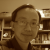Chaos gameChaos game - square fractal

Chaos game is a mathematical way to generate fractals. It has a simple algorithm, which (1) starts from a radom point with a polygon, then (2) randomly selects a vertice, (3) plots a new point midway between the starting point and the selected vertice. The ploted mid point then becomes the step (1) and reiterates the steps as many times as you like to generate a geometric figure. The example below in this blog uses a square as the polygon to generate a chaos game square fractal.